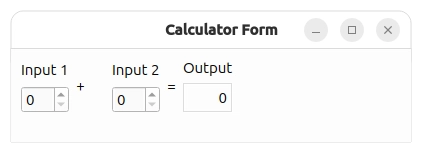# Calculator Form/Multiple Inheritance

Using a form created with Qt Designer in an application.

The Multiple Inheritance Example shows how to use a form created with Qt Designer in an application by subclassing both QWidget and the user interface class, which is `Ui::CalculatorForm`.To subclass the `calculatorform.ui` file and ensure that `qmake` processes it with the `uic`, we have to include `calculatorform.ui` in the `.pro` file, as shown below:

```QT += widgets

SOURCES = calculatorform.cpp main.cpp
FORMS = calculatorform.ui```

When the project is compiled, the `uic` will generate a corresponding `ui_calculatorform.h`.

#### CalculatorForm Definition

In the `CalculatorForm` definition, we include the `ui_calculatorform.h` that was generated earlier.

`#include "ui_calculatorform.h"`

As mentioned earlier, the class is a subclass of both QWidget and `Ui::CalculatorForm`.

```class CalculatorForm : public QWidget, private Ui::CalculatorForm
{
Q_OBJECT

public:
explicit CalculatorForm(QWidget *parent = nullptr);

private slots:
void on_inputSpinBox1_valueChanged(int value);
void on_inputSpinBox2_valueChanged(int value);
};```

Two slots are defined according to the automatic connection naming convention required by `uic`. This is to ensure that QMetaObject's auto-connection facilities connect all the signals and slots involved automatically.

#### CalculatorForm Implementation

In the constructor, we call `setupUi()` to load the user interface file. Note that setupUi is a method of `Ui::CalculatorForm`.

```CalculatorForm::CalculatorForm(QWidget *parent)
: QWidget(parent)
{
setupUi(this);
}```

We include two slots, `on_inputSpinBox1_valueChanged()` and `on_inputSpinBox2_valueChanged()`. These slots respond to the valueChanged() signal that both spin boxes emit. Whenever there is a change in one spin box's value, we take that value and add it to whatever value the other spin box has.

```void CalculatorForm::on_inputSpinBox1_valueChanged(int value)
{
outputWidget->setText(QString::number(value + inputSpinBox2->value()));
}

void CalculatorForm::on_inputSpinBox2_valueChanged(int value)
{
outputWidget->setText(QString::number(value + inputSpinBox1->value()));
}```

#### `main()` Function

The `main()` function instantiates QApplication and `CalculatorForm`. The `calculator` object is displayed by invoking the show() function.

```int main(int argc, char *argv[])
{
QApplication app(argc, argv);
CalculatorForm calculator;
calculator.show();
return app.exec();
}```

There are various approaches to include forms into applications. The Multiple Inheritance approach is just one of them. See Using a Designer UI File in Your Application for more information on the other approaches available.

Example project @ code.qt.io